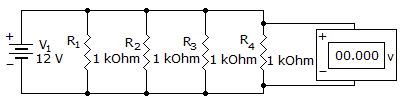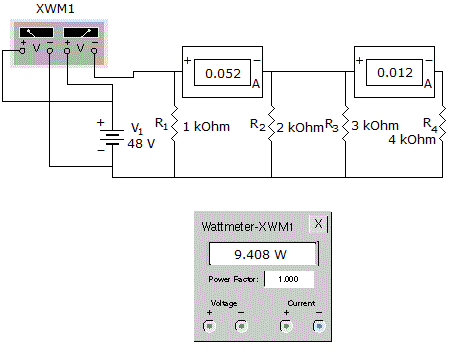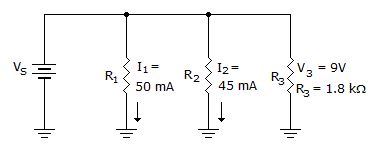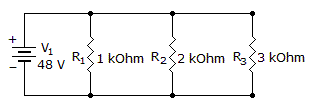# Electronics - Parallel Circuits

### Exercise :: Parallel Circuits - General Questions

16.

The voltage read by the voltmeter will be:A. 24 V B. 12 V C. 6 V D. 4 V

Explanation:

No answer description available for this question. Let us discuss.

17.

Which component is of the wrong value?A. R1 B. R2 C. R3 D. R4

Explanation:

No answer description available for this question. Let us discuss.

18.What does I3 equal in the given circuit?

 A. 5 mA B. 45 mA C. 50 mA D. 100 mA

Explanation:

No answer description available for this question. Let us discuss.

19.

What is the total power of the circuit?A. 2.2 W B. 4.2 W C. 6.2 W D. 8.2 W

Explanation:

No answer description available for this question. Let us discuss.

20.

What are the individual values of two parallel resistors whose RT is 8 ohms, when one has double the resistance of the other?

 A. 2.7 ohms and 5.3 ohms B. 8 ohms and 16 ohms C. 10 ohms and 20 ohms D. 12 ohms and 24 ohms